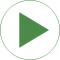# Information

This information is part of the Modelica Standard Library maintained by the Modelica Association.

Synchronous machine with permanent magnets at no-load, driven with constant nominal speed.

You may check the terminal voltage = VsOpenCircuit (shown by the length of the space phasor) and the frequency = fsNominal.

Additionally, you may check the phase shift of the stator voltages with respect to the mechanical shaft angle:

• If the shaft angle starts at (pi + 0*pi/3)/p, the flux linkage through phase 1 is at the maximum and therefore this phase voltage starts at 0.
• If the shaft angle starts at (pi + 2*pi/3)/p, the flux linkage through phase 2 is at the maximum and therefore this phase voltage starts at 0.
• If the shaft angle starts at (pi + 4*pi/3)/p, the flux linkage through phase 3 is at the maximum and therefore this phase voltage starts at 0.

Note that the angle of the voltage space phasor is pi/2 behind the angle of the hall sensor, i.e. after a rotation of the shaft by pi/2/p the flux linkage of phase 1 is zero and the induced voltage a maximum.

# Parameters (1)

smpmData Value:

# Components (10)

smpmData smpm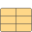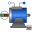Type: SM_PermanentMagnet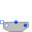Type: TerminalBox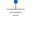Type: Ground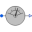Type: PotentialSensor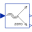Type: ToSpacePhasor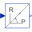Type: ToPolar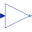Type: Gain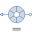Type: HallSensor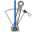Type: ConstantSpeed